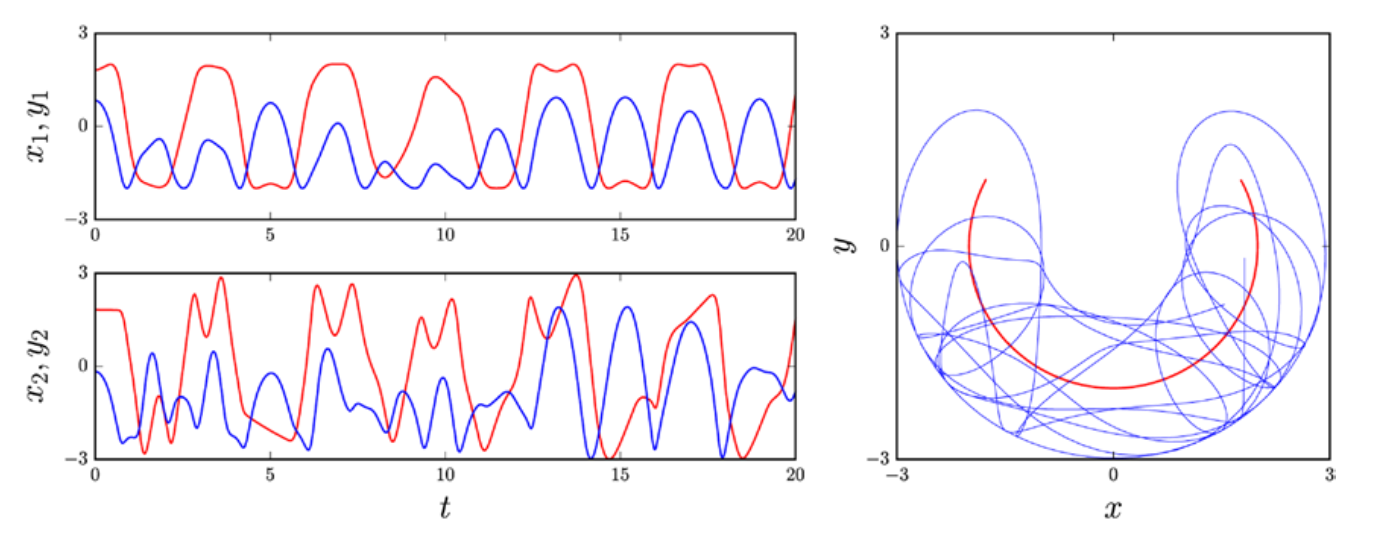## 常微分方程

ODE 总是可以重写为一阶常微分方程组。具体而言 n 阶 ODE $\frac{d^ny}{dx^n} = g(x, y, \frac{dy}{dx},...,\frac{d^{n-1}y}{dx^{n-1}})$，可以通过引入 n 个新函数以标准形式显式d地写为 $y_1 = y, y_2 = \frac{dy}{dx}, ..., y_n = \frac{d^{n-1}y}{dx^{n-1}}$。这给出了以下一阶常微分方程组：

## 符号求解 ODE

SymPy 提供了一个通用的 ODE 求解工具 sympy.dsolve，它能够找到许多基本 ODE 的解析解。 sympy.dsolve 函数会尝试自动对给定的 ODE 进行分类，尝试使用各种技术来求解给定 ODE。我们可以给 dsolve 函数一些提示，这可以引导它到最合适的求解方法。虽然 dsolve 可以用来符号化地求解许多简单的 ODE，但我们将在后面看到（值得记住的是）大多数 ODE 不能解析地求解。 ODE 符号解的典型例子是一阶或二阶 ODE 的求解，以及具有较少未知函数的一阶线性常微分方程的求解。如果 ODE 具有特殊的对称性，或其他特性，如可分离、具有常数系数或存在已知解析解的特殊形式，则它也有很大帮助。虽然这些 ODE 类型是例外情况和特殊情况，但这些 ODE 有许多重要的应用，对于这些情况，SymPy 的 dsolve 可能是对传统分析方法的非常有用的补充。在本节中，我们将探讨如何使用 SymPy 及其 dsolv e函数来解简单但常见的 ODE。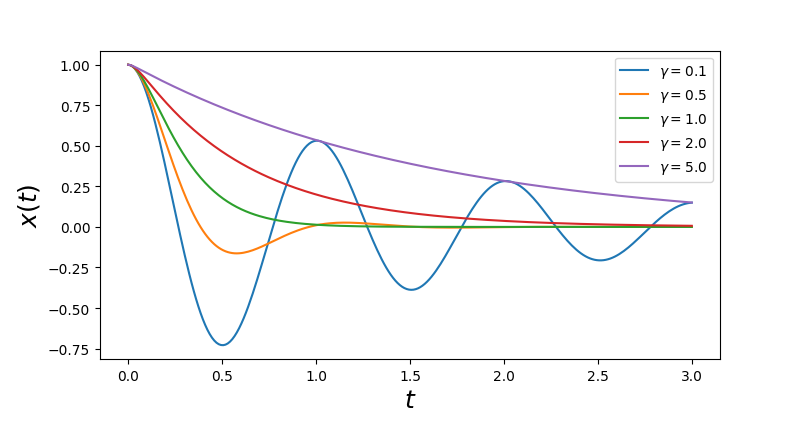### 方向场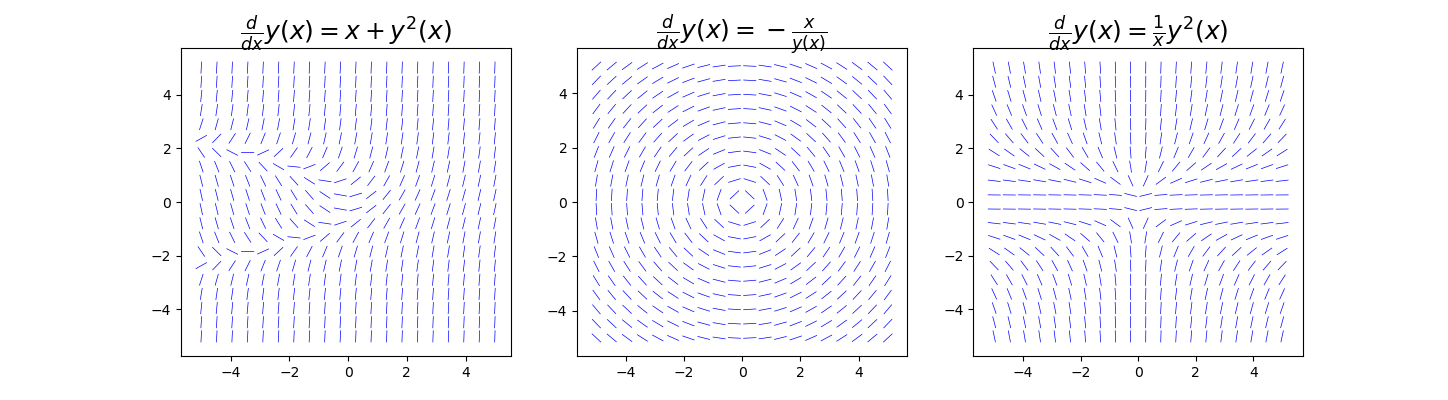## 数值方法求解 ODE

### 使用 SciPy 求解 ODE 数值微分

odeint 函数有三个强制性参数：用于评估标准形式 ODE 右侧的函数、指定未知函数初始条件的数组（或标量）以及具有自变量的数组，其中未知函数要被计算。ODE 右侧的函数有两个强制参数和任意数量的可选参数。必需的参数是向量 y(x) 的数组（或标量）以及 x 的值。再次考虑标量 ODE $y^{'}(x) = f(x, y(x)) = x + y(x)^2$。为了能够再次绘制这个 ODE 的方向场，这次与使用 odeint 进行数值积分得到的特解一起，我们首先定义构造 $f(x, y(x))$ 符号表达式所需的 SymPy 符号：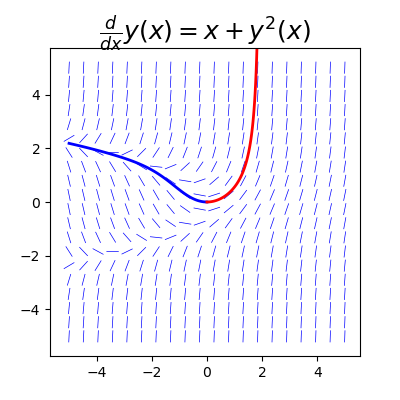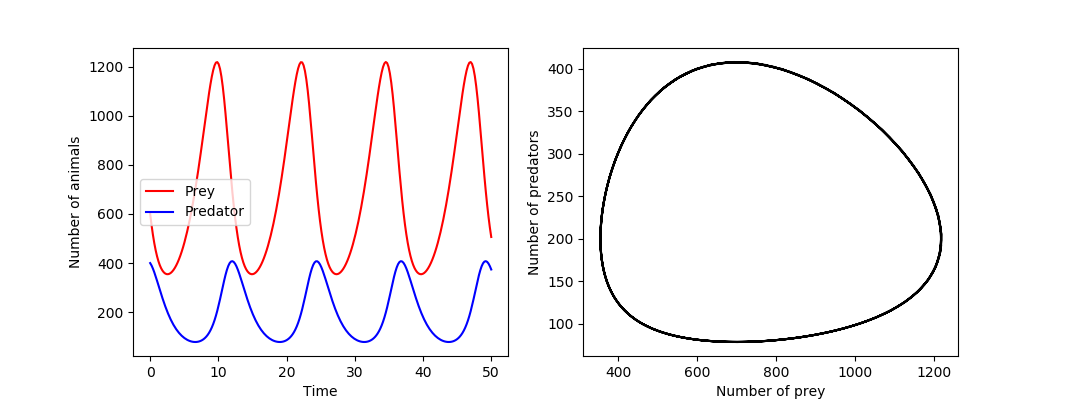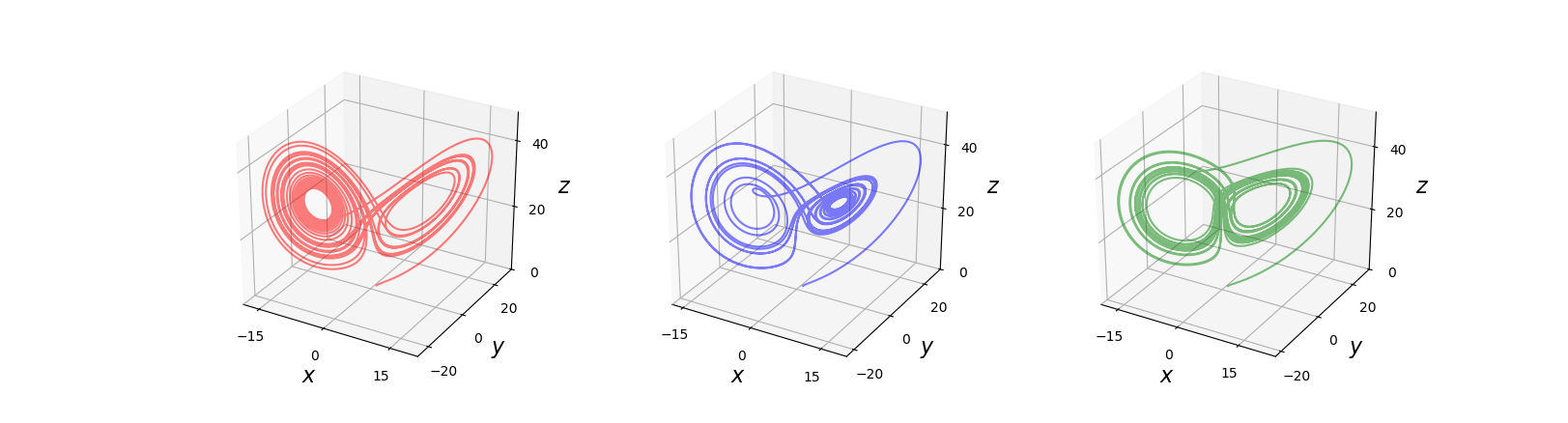integrate.ode类可以与不同求解器一起工作，并且对每个求解器都有特定的选项。为了说明如何使用 integrate.ode 接口，我们首先看看以下几组耦合二阶 ODE：

integrate.odeintintegrate.ode 之间的主要区别是从这一点开始。我们现在需要创建类 integrate.ode 的实例，将 ODE 函数 f 作为参数传递，而不是调用 odeint 函数：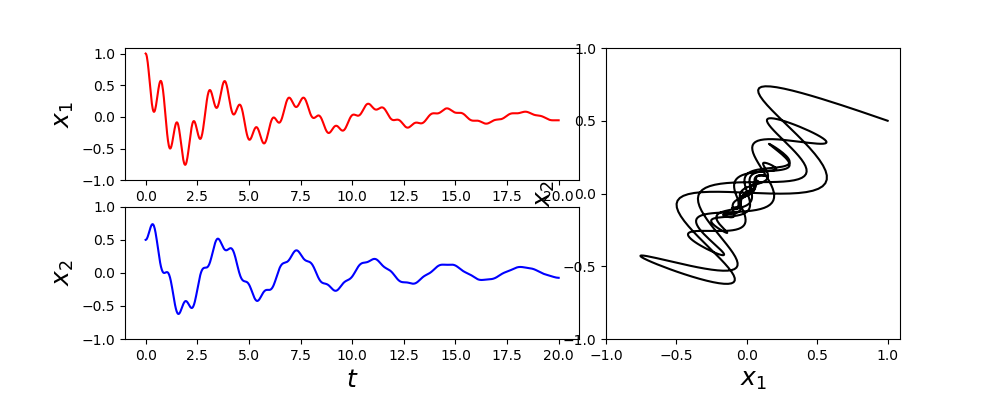ODE 的解现在存储在数组 y 中。当可视化该解时，绘制摆在 x-y 平面中的位置比绘制它们的角度偏转更直观。角变量 $\theta_1$$\theta_2$ 与 x 和 y 坐标之间的转换为：$x_1=l_1sin\theta_1$$y_1=l_1cos\theta_1$$x_2=x_1 + l_2sin\theta_2$$y_2 = y_1 + l_2cos\theta_2$